## Меню

Главная
Финансы деньги и налоги
Издательское дело и полиграфия
Новейшая история политология
Топики по английскому языку
Языкознание филология
Химия
Экология и охрана природы
Естествознание
Искусство и культура
Культурология
Хозяйственное право
Иностранные языки и языкознание
История и исторические личности
Коммуникации связь цифровые приборы и радиоэлектроника
Товароведение
Транспорт
Экология охрана труда
Банковское и биржевое дело
Коммуникации и связь
Конституционное право
Этика

##### Научно-образовательный портал W-10.RU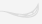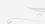Главная

# How Many Physical Constants are Truly Fundamental?

Internal Structure of a Proton and New Method of Receiving of Energy Dr. N.V. Kosinov, V. I. Garbaruk INTRODUCTION

INTERNAL STRUCTURE of a PROTON

In articles [2 -5] the steps in a direction of creation of the theory of internal structure of elementary particles are undertaken. The relation reflecting the law of formation of structure of an elementary particle is received: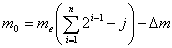(1)

Where: n = 2,3...; me - mass of a electron ; j = 1 for neutral particles; j = 0 for particles having a charge.

From the formula it is visible, that the law of genesis of structure of substance has binary character. Binarity is being kept on each step of structurization of material. Binarity in the laws of genesis of structure reflects, that the charging conjugate particles participate in formation of other particles. The formula reflects dynamics of genesis of structure, according to which a formation of structure of material particles is taking place.

The relations for energies are described by the following mathematical formulas [2 - 5]: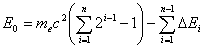(2)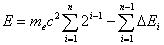(3)

The formula (2) is applicable for definition of energy of neutral particles, the formula (3) is applicable for definition of energy of charged particles. Each of formulas (2) and (3) reflects the certain discrete series of power levels, at their correspondence to sequence of material formations in dynamics of genesis of structure. Formulas (2) and (3) show that the energy participating in formation of particles is divided into two components. The first component represents total rest energy of material formations participating in formation of a new particle. So, for neutral material formations, it is presented as: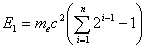(4)

The second component is presented by summands, which determine size of binding energy: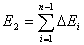(5)

The relations (2) and (3) reflect discrete character of internal energy of elementary particles.

In  it is shown, that the internal structure of a proton represents fractal construction.

Fractal, revealed in structure of elementary particles, reflects the determinate process of genesis of structure. For fractal of a proton the alternation of symmetric and asymmetric branches of treelike structure is characteristic. The discovering of a concrete kind of fractal, reflecting law of genesis of structure of a proton, allows to receive the quantitative characteristics of elementary particles by a Яalculated way. In  the quantitative characteristics of fractal structures of elementary particles are received and the mathematical description of fractal of a proton is found. The mathematical relation for an elementary cell of fractal of a proton is presented as: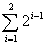On the bases of the formula for an elementary cell of fractal the generalized mathematical relation describing completed fractal designs of internal structure of elementary particles is received. This mathematical relation is presented as: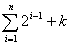,

Where: k = 0 for nucleuses, k = 1 for atoms, k = -1 for neutral particles.

From an above mentioned relation, at n = 11, we receive the formula describing fractal of a proton: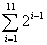. (6)

The formula for fractal of hydrogen will look like: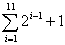.

Considering law of genesis of structure of a proton, it is visible to see reflection of self-similarity in its internal structure on various steps of genesis of structure. On each step of formation of structure of a particle the uniform algorithm of shape formation, resulting in original topology of a proton is traced. Fractal of a proton is described by the following topological formula :

Pp =2(2(2(2(2(2(2(2(2(2+1)+1)+1)+1)+1)+1)+1)+1)+1)+1 (7)

The graphic image of the topological formula of a proton represents treelike fractal design. The process of formation of a proton is realized as a result of the consecutive acts of energy saturation of vacuum [2, 3, 4]. The internal structure of a proton is formed by system of consecutive enclosures based on uniform algorithm. On each step of genesis of structure of a proton the fractal substructure repeats fractal of the previous step of structurization. The research of fractal of a proton shows that the internal structure of a proton has brightly expressed quantization and hierarchy structure. In this hierarchy each subsystem is created on the same image: each bigger part of structure in accuracy repeats a smaller part of structure. Thus spatial arrangement at formation of internal structure of a proton is displaied. According to the formulas (4), (6), (7) there is a hierarchical discrete series of internal levels of energy of a proton. Proceeding from the law of preservation of energy, it is visible, that for internal structure of a proton the hierarchy of characteristic frequencies is taking place. As a result, spatial arrangement exists alongside with temporary arrangement. For internal structure of a proton each part of the higher order is constructed by means of unification of two structures of the lower order.

It results in the special grid of characteristic of internal frequencies being created by a principle of DOUBLING of the period.

It is demonstrated by the topological formula of a proton (7). Recursive algorithm is applicable for discribing of dynamics of genesis of structure of a proton and topological formula of a proton in language of algorithms . Recurcity is copying small in large and large in small, keeping a uniform principle of construction of structure of a proton. The formulas of genesis of structure of elementary particles and topological formula of a proton have allowed to receive by theoretical calculation a fundamental constant mp/me, that specifies experimental confirmation of the theory [5, 7, 8, 10].

Besides of, these formulas have allowed to find the mechanism crucial for steadiness of a proton, that opens new methods of receiving of energy. It will be shown below.

ENERGY OF VACUUM AND ENERGY OF A PROTON

In the quantum theory is proved, that the minimal possible energy of oscillator cannot be less than 0.5hl (6). These halves at a wide range of waves give very high level of energy of vacuum. It, so-called, zero - point energy of electromagnetic fluctuations. Experimentally energy of vacuum is observed in Lemba-Ryzerford effect and in Kazimir effect. Density of energy of vacuum is defined by relation (6) :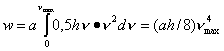,

where: h -Planck`s constant, a - coefficient, h - frequency .

From here follows, that the energy of vacuum can be very large. According to R. Feynman and G. Whiller, the energy potential of vacuum is so huge, that " in vacuum enclosed in volume of an ordinary electrical bulb, energy such plenty, that it would suffice to boil all oceans on the Earth ".

However, due to high symmetry of vacuum, the direct access to this energy is very inconvenient. As a result, being found, in essence, among ocean of energy, the mankind is compelled to use only traditional methods of receiving of energy based on burning natural energy carriers.

Nevertheless, at infringement of symmetry of vacuum the access to ocean of energy is possible.

The modern methods of receiving of energy can schematically be presented as:

C + O2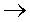CO2 + 0,0046 MeV,

235U0,85 MeV / nucleon + radioactive waste,

D + T4He + 17,6 MeV .

When analysing these methods of receiving of energy, it is possible to see, that a final product in circuit of power transformations is the substance. And, this final substance becomes, as a rule, more dangerous to biosphere, than initial energy carriar. The world already get used to an idea, that for receiving of energy is necessary to have an effect on substance and at a final stage also to receive substance. It concerns energy power based on burning of natural fuel, both to atomic engineering, and to thermonuclear synthesis. Thus cost of received energy remains high, and waste are very dangerous to biosphere. For such methods of receiving of energy the formulation suits: " substance in the beginning of energy transformations - substance in the end of energy transformations ". The task is to find new methods of receiving of energy, free from drawbacks of the traditional scheme. The new scheme of energy transformations should look as: " Substance in the beginning of energy transformations - energy in the end ". Then at a final stage of energy transformations there will be no substance, dangerous to biosphere. It is possible only in case when there are no reactions of synthesis in the circuit of energy transformations, and instead of them the reactions destructurization of substance are realized. It is possible in medium of energy saturated vacuum, where there is an infringement of its symmetry. As a result, there is no direct access to energy of vacuum, but the exchange of energy of vacuum on energy contained in substance is carried out. The transformation of substance to energy will allow considerably to increase quantity of received energy and to make process of receiving of energy by ecologically clean. The new scheme of energy transformations can be realized at presence of a high level of excitation energy of vacuum and influence of this energy on substance. For this purpose a proton ideally suitable as "fuel".

In second half of 20-th century the theoretical physics has come to a conclusion about an opportunity of disintegration of a proton [6,11]. The disintegration of a proton represents the very tempting phenomenon for the purpose of receiving of vacuum energy. Let's confirm it by concrete calculations. In [3,7,8,10] the physical constants hu, tu, lu,, П, concerning vacuum are found. These constants have allowed to receive mass of a proton by mathematical calculation . The formulas for mass of a proton, with application of universal superconstants of vacuum hu, tu, lu,, П, directly follow from parities(ratio) (1) - (7).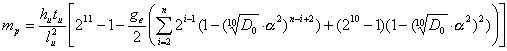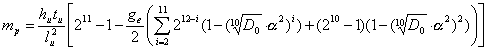Let's note, that from the above mentioned formulas, major fundamental physical constant mp/me [5,8,10] directly follows. Component, which represents binding energy is entering into the formulas of mass of a proton. This energy determines a degree of steadiness of a particle. We have the opportunity to calculate its value. The formula for definition of binding energy of a proton is presented as :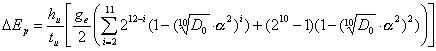(8)

The value of binding energy has been calculated on this formula, is equal 107,74 лev (? 108 лeV) and makes about 11,5 % from rest energy of a proton. Thus the major characteristic of a proton is determined, knowledge of which is the key moment for realization of a new method of receiving of energy. If a proton is receiving extra energy, such, that it exceeded binding energy (? 108 MeV), it becomes unsteady and disintegrates on light particles having very small time of life. It is possible at the certain level of energy saturation of vacuum in a local zone of space, where there is a proton. The new method of receiving of energy is based on this principle.

The basic stage of energy transformations in a new method of receiving of energy can be presented as:

p+ + 108 MeV938 MeV

Here instead of reaction of synthesis of substance, energy saturation of a proton is realized, that results in it destructurization. On Fig.1 the complete scheme of energy transformations in a new method of receiving of energy is shown.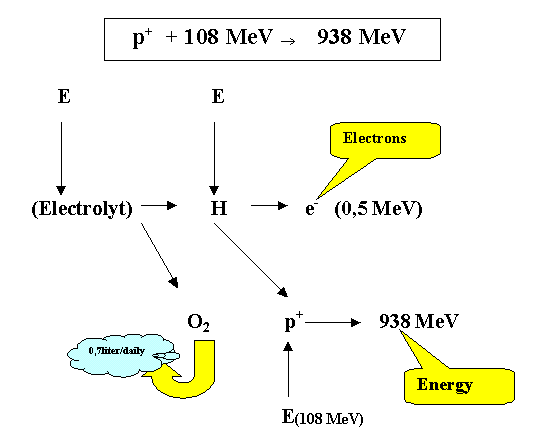Fig.1. The scheme of energy transformations in a new method of receiving of energy.

The calculations show, that the binding energy equal to 107,74 лeV and contains 10 components:

107,74 лeV = 54,9 + 20,35 + 13,35 + 8,23 + 4,84 + 2,84 + 1,62 + 0,87 + 0,48 + 0,26 (лeV).

Accordingly, the power influence on a proton should be carried out by quantums of energy and should be correspond to 10steps. As all elementary particles, on which the proton disintegrates, are unsteady, such scheme does not result in occurrence of residual substance at a final stage of energy transformations [12, 13]. It makes a method by ecologically clean. Other advantage of a new method is the high unprecedented output energy. As it is visible from the formulas for mass of a proton and from a relation (8), the specific energy more than in one a thousand times exceeds opportunities of atomic power and in dozens of times exceeds opportunities of thermonuclea synthesis, remaining at that by ecologically clean method. In this method the influence on nucleuses of atoms of hydrogen is carried out. The influence is carried out in a local zone of space at energy saturation of vacuum in medium of electro Яonductivity of a liquid. The method allows to receive thermal and electrical energy. The calculations show that energy coefficient is equal to K = 4,8 - 8,6. Consuming material is electrolyt. Consumption of electrolyt is 1 gramme on 2500 KW T hour of energy. When using of a electrolyt on a water basis the residual substance - oxygen will be formed. For the generator by capacity of 100 KW the eduction of O2 makes very insignificant quantity equal to 0,25 cub.m per one year.

The stated above approach usen idea of infringement of steadiness of a proton, can find application for utilization of various waste of manufacture in an industry and power. The influence on substance with the purpose of it destructurization can become the universal and effective tool of ensuring of ecological safety of manufacture and transformation of waste of manufacture into a thermal energy. It essentially changes a sight for existing types and classes of energy carriers and will allow to consider even dangerous waste as potential energy carriers.

REFERENCE

1. л.Jacob, P.Landshoff, Internal structure of a proton, UFN, vol. 133, pub. 3, 1981.

2. N. Kosinov, Emanation of substance by vacuum and law of genesis of structure, Physical vacuum and nature, N1, 1999.

3. N. Kosinov, Physical vacuum and gravitation. Physical vacuum and nature, N4, 2000.

4. N. Kosinov, Wireless transmission of energy, Idea. N2, 1994, p.g. 221 -227.

5. N. Kosinov, An origin of a proton. Physical vacuum and nature, N3, 2000.

6. Y. Zeldovich, The theory of vacuum can solve a problem of Яosmology, UFN, vol.133,pub.3, 1981.

7. N. Kosinov, Five Fundamental Constants of Vacuum, Lying in the Base of all Phisical Laws, Constants and Formulas, Physical Vacuum and Nature, ¦4, 2000.

8. N. Kosinov, Z. Kosinova, Connection of Gravitational Constant G and Planck Constant h, 51st International Astronautical Congress 2-6 Oct. 2000/Rio de Janeiro, Brazil.

9. A. Anisimov, Computer science. Creativity.Recursity, K., Naukova dumka, 1988.

10. N. Kosinov, Five universal physical constants, lying in the base of all fundamental constants, laws and formulas of physics , The sixth International conference ? Modern problems of natural sciences ¦, The program and theses.S.-Petersburg, August, 2000.

11. ю. Sacharov, Infringement of CP-invariancy, C - symmetry and baryonous asymmetry of the universe, The letters in GETF,vol.5, 1967, p.g.33-35.

12. N. Kosinov, V. Garbaruk, Vacuum origin of Electron , Physical vacuum and nature, N1, 1999.

13. N.Kosinov, V.Garbaruk ,Vacuum origin of Electron and Positron". Physical Vacuum and Nature, N4, 2000.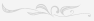## Поиск

Расширенный поиск